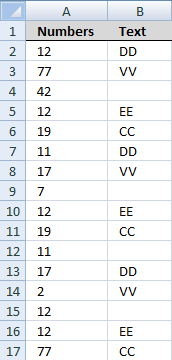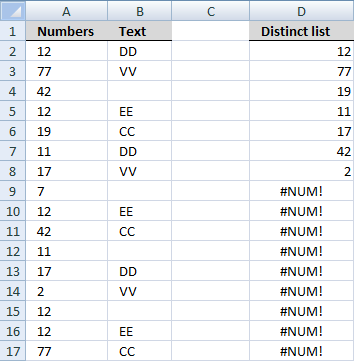Author: Oscar Cronquist Article last updated on February 06, 2018

Question: I want a unique list to be created from a column where an adjacent column has text cell value?Array formula in D3:

=INDEX(\$A\$2:\$A\$17, MATCH(0, COUNTIF(\$D\$1:D1, \$A\$2:\$A\$17)+(ISTEXT(\$B\$2:\$B\$17)=FALSE), 0))

Recommended articles

Vlookup – Return multiple unique distinct values

Ahmed Ali asks: How to return multiple values using vlookup in excel and removing duplicates? I have tried the formula […]

Unique distinct list sorted alphabetically based on a condition

The array formula in cell E6 filters values in column C based on value in cell E3, the output is […]

Create a unique distinct list based on criteria

The regular formula in cell E6 extracts unique distinct values from column B based on the corresponding number in column […]

How to create an array formula

1. Copy array formula (Ctrl + c)
2. Double click cell D2
3. Paste array formula (Ctrl + v)
4. Press and hold Ctrl + Shift
5. Press Enter

Recommended article

How to enter an array formula

Array formulas allows you to do advanced calculations not possible with regular formulas.

#### Explaining formula in cell D3

Step 1 - Check if adjacent values are not text values

ISTEXT(\$B\$2:\$B\$17)=FALSE

becomes

ISTEXT({"DD";"VV";0;"EE"; "CC";"DD";"VV";0;"EE";"CC";0; "DD";"VV";0;"EE";"CC"})=FALSE

becomes

{TRUE; TRUE;FALSE;TRUE;TRUE; TRUE;TRUE;FALSE;TRUE; TRUE;FALSE;TRUE;TRUE;FALSE;TRUE;TRUE}=FALSE

and returns

{FALSE;FALSE; TRUE;FALSE;FALSE; FALSE;FALSE;TRUE;FALSE;FALSE; TRUE;FALSE;FALSE; TRUE;FALSE;FALSE}

Step 2 - Check if value is unique

COUNTIF(\$D\$1:D1, \$A\$2:\$A\$17)+(ISTEXT(\$B\$2:\$B\$17)=FALSE)

becomes

COUNTIF(\$D\$1:D1, \$A\$2:\$A\$17)+(ISTEXT(\$B\$2:\$B\$17)=FALSE)

becomes

{0;0;0;0;0;0;0;0;0;0;0;0;0;0;0;0}+(ISTEXT(\$B\$2:\$B\$17)=FALSE)

becomes

{0;0;0;0;0;0;0;0;0; 0;0;0;0;0;0;0}+{FALSE;FALSE;TRUE; FALSE;FALSE;FALSE;FALSE;TRUE;FALSE; FALSE;TRUE;FALSE;FALSE; TRUE;FALSE;FALSE}

and returns

{0;0;1;0;0;0;0;1;0;0;1;0;0;1;0;0}

How to use the COUNTIF function

Counts the number of cells that meet a specific condition.

Step 3 - Find relative position

MATCH(0, COUNTIF(\$D\$1:D1, \$A\$2:\$A\$17)+(ISTEXT(\$B\$2:\$B\$17)=FALSE), 0)

becomes

MATCH(0, {0;0;1;0;0;0;0;1;0;0;1;0;0;1;0;0}, 0)

and returns 1.

How to use the MATCH function

Identify the position of a value in an array.

Step 4 - Return value

INDEX(\$A\$2:\$A\$17, MATCH(0, COUNTIF(\$D\$1:D1, \$A\$2:\$A\$17)+(ISTEXT(\$B\$2:\$B\$17)=FALSE), 0))

becomes

INDEX(\$A\$2:\$A\$17, 1)

and returns 12 in cell D3.

How to use the INDEX function

Gets a value in a specific cell range based on a row and column number.